# On some inequalities of a certain class of analytic functions

## Abstract

The aim of this paper is to study the properties of a subclass of analytic functions related to p-valent Bazilevic functions by using the concept of differential subordination. We investigate some results concerned with coefficient bounds, inclusion results, radius problem, covering theorem, angular estimation of a certain integral operator, and some other interesting properties.

MSC:30C45, 30C50.

## 1 Introduction and preliminaries

Let $A p$ be the class of analytic functions

$f(z)= z p + ∑ n = p + 1 ∞ a n z n ( p ∈ N = { 1 , 2 , 3 , … } ) ,$
(1.1)

defined in the open unit disc $E={z:|z|<1}$. A function $f∈ A p$ is a p-valent starlike function of order ρ if and only if

$Re z f ′ ( z ) f ( z ) >ρ,0≤ρ

This class of functions is denoted by $S p ∗ (ρ)$. It is noted that $S p ∗ (0)= S p ∗$. If $f(z)∈ A p$ satisfies

$|arg z f ′ ( z ) p f ( z ) |< π η 2$
(1.2)

for some $η∈(0,1]$ and for all $z∈E$, then the function f is called strongly starlike p-valent of order η in E. We denote this class by $S ˜ p ∗ (η)$. Let $f 1 (z)$ and $f 2 (z)$ be analytic in E. We say $f 1 (z)$ is subordinate to $f 2 (z)$, written $f 1 ≺ f 2$ or $f 1 (z)≺ f 2 (z)$, if there exists a Schwarz function $w(z)$, $w(0)=0$, and $|w(z)|<1$ in E, then $f 1 (z)= f 2 (w(z))$. A function f in $A p$ is said to belong to the class $S p ∗ [A,B]$, $−1≤B, if and only if

$z f ′ ( z ) f ( z ) ≺p 1 + A z 1 + B z ,z∈E.$

For $p=1$, we obtain the class $S ∗ [A,B]$ of Janowski starlike functions. Janowski functions have extensively been studied by several researchers; see for example . It is clear that $f∈ S p ∗ [A,B]$ if and only if

$| z f ′ ( z ) f ( z ) −p 1 − A B 1 − B 2 |
(1.3)

and

$Re z f ′ ( z ) f ( z ) >p 1 − A 2 (B=−1,z∈E).$

A function $f∈ A p$ is p-valent Bazilevic function of type $(α,β)$ and order ρ if and only if

$Re z f ′ ( z ) f ( z ) ( f ( z ) g ( z ) ) α ( f ( z ) z p ) i β >ρ,0≤ρ

where $α≥0$, $β∈R$ and $g∈ S p ∗$. For $p=1$ and $ρ=0$, this class was introduced by Bazilevic and these functions are univalent for $α≥0$, $β∈R$. This class of functions is studied by many authors; for some details, see .

Using this concept, we generalize and define a subclass of p-valent Bazilevic functions of type $(α,β)$ as follows.

Definition 1.1 A function $f∈ M p (α,β,μ,A,B)$ if it satisfies the condition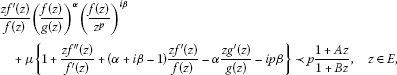where $α≥0$, $μ>0$, $g∈ S p ∗$, $−1≤B and β is any real.

We have the following special cases.

1. (i)

For $β=0$, we have the subclass of Bazilevic functions defined by Patel .

2. (ii)

For $β=0$, $p=1$, $g(z)=z$, $A=1−2ρ$, $B=−1$, we obtain the subclass of Bazilevic functions defined in .

For $A=1−2ρ$, $B=−1$, we have the following subclass of analytic functions.

Definition 1.2 A function $f∈ B p (α,β,ρ)$ if it satisfies the conditionwhere $α≥0$, $μ>0$, $g∈ S p ∗$, $0≤ρ<1$, β is any real and $z∈E$. In other words, a function $f∈ B p (α,β,ρ)$ if it satisfies the condition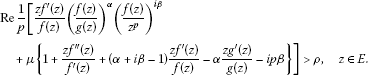We need the following definition and lemmas which will be used in our main results.

Definition 1.3 Let $Ψ: C 2 ×E→C$ be analytic in a domain D and h be univalent in E. If p is analytic in E with $(p(z),z p ′ (z);z)∈D$ when $z∈E$, then we say that p satisfies a first-order differential subordination if

$Ψ ( p ( z ) , z p ′ ( z ) ; z ) ≺h(z),z∈E.$
(1.4)

The univalent function q is called dominant of the differential subordination (1.4) if $p≺q$ for all p satisfies (1.4). If $q ˜ ≺q$ for all dominants of (1.4), then we say that $q ˜$ is the best dominant of (1.4).

Lemma 1.4 ()

If $−1≤B, $λ>0$ and the complex number γ satisfies $Re{γ}≥−λ(1−A)/(1−B)$, then the differential equation

$q(z)+ z q ′ ( z ) λ q ( z ) + γ = 1 + A z 1 + B z ,z∈E,$

has a univalent solution in E given by

$q(z)={ z λ + γ ( 1 + B z ) λ ( A − B ) / B λ ∫ 0 z t λ + γ − 1 ( 1 + B t ) λ ( A − B ) / B d t − γ λ , B ≠ 0 , z λ + γ e λ A z λ ∫ 0 z t λ + γ − 1 e λ A t d t − γ λ , B = 0 .$

If $h(z)=1+ c 1 z+ c 2 z 2 +⋯$ is analytic in E and satisfies

$h(z)+ z h ′ ( z ) λ h ( z ) + γ ≺ 1 + A z 1 + B z ,z∈E,$

then

$h(z)≺q(z)≺ 1 + A z 1 + B z ,$

and $q(z)$ is the best dominant.

Lemma 1.5 ()

Let ε be a positive measure on $[0,1]$. Let g be a complex-valued function defined on $E×[0,1]$ such that $g(⋅,t)$ is analytic in E for each $t∈[0,1]$ and $g(z,⋅)$ is ε-integrable on $[0,1]$ for all $z∈E$. In addition, suppose that $Reg(z,t)>0$, $g(−r,t)$ is real and $Re{1/g(z,t)}≥1/g(−r,t)$ for $|z|≤r<1$ and $t∈[0,1]$. If $g(z)= ∫ 0 1 g(z,t)dε(t)$, then $Re{1/g(z)}≥1/g(−r)$.

Lemma 1.6 ([, Chapter 14])

Let $a 1$, $b 1$ and $c 1 ≠0,−1,−2,…$ be complex numbers. Then, for $Re c 1 >Re b 1 >0$,Lemma 1.7 ()

Let $−1≤ B 1 ≤ B 2 < A 2 ≤ A 1 ≤1$. Then

$1 + A 2 z 1 + B 2 z ≺ 1 + A 1 z 1 + B 1 z .$

Lemma 1.8 ()

Let F be analytic and convex in E. If $f,g∈ A p$ and $f,g≺F$, then

$μf+(1−μ)g≺F,0≤μ≤1.$

Lemma 1.9 ()

Let $f(z)= ∑ k = 0 ∞ a k z k$ be analytic in E and $F(z)= ∑ k = 0 ∞ b k z k$ be analytic and convex in E. If $f≺F$, then

$| a k |≤| b 1 |(k∈N).$

Lemma 1.10 ()

Let $h(z)=1+ d 1 z+ d 2 z 2 +⋯$ be analytic in E and $h(z)≠0$ in E. If there exists a point $z 0 ∈E$ such that $|argh(z)|< π 2 η$ ($|z|<| z 0 |$) and $|argh( z 0 )|= π 2 η$ ($0<η≤1$), then we have $z 0 h ′ ( z 0 ) h ( z 0 ) =ikη$, where

${ k ≥ 1 2 ( x + 1 x ) , when arg h ( z 0 ) = π 2 η , k ≤ − 1 2 ( x + 1 x ) , when arg h ( z 0 ) = − π 2 η ,$

and $( h ( z 0 ) ) 1 / η =±ix$ ($x>0$).

Lemma 1.11 Let $g∈ S ∗ [A,B]$. Then the function

$G(z)= [ c + α + i β z c + i β ∫ 0 z t c + i β − 1 g α ( t ) d t ] 1 α$
(1.5)

belongs to $S ∗ [A,B]$ for $c≥−α 1 − A 1 − B$.

Proof is straightforward by using Lemma 1.4.

Throughout this paper, $α≥0$, $β∈R$, $μ>0$, and $−1≤B unless otherwise stated.

## 2 Main results

Theorem 2.1 If $f∈ M p (α,β,μ,A,B)$, then

$z f ′ ( z ) p f ( z ) ( f ( z ) g ( z ) ) α ( f ( z ) z p ) i β ≺q(z),$
(2.1)

where $q(z)= μ p Q ( z )$ and

$Q(z)={ ∫ 0 1 t p μ − 1 ( 1 + B z t 1 + B z ) p μ ( A − B ) / B d t , B ≠ 0 , ∫ 0 1 t p μ − 1 e p μ ( t − 1 ) A z d t , B = 0 .$
(2.2)

In hypergeometric function form,

$q(z)={ [ 2 F 1 ( 1 , p μ ( 1 − A B ) ; p μ + 1 ; B z B z + 1 ) ] − 1 , B ≠ 0 , [ 1 F 1 ( 1 , p μ + 1 ; − p μ A z ) ] − 1 , B = 0 ,$
(2.3)

and if $A<− μ B p$, $−1≤B<0$, then $M p (α,β,μ,A,B)⊂ B p (α,β,ρ)$, where

$ρ=p { 2 F 1 ( 1 , p μ ( 1 − A B ) ; p μ + 1 ; B B − 1 ) } − 1 .$
(2.4)

This result is best possible.

Proof Let

$h(z)= z f ′ ( z ) p f ( z ) ( f ( z ) g ( z ) ) α ( f ( z ) z p ) i β ,$

where $h(z)$ is analytic in E with $h(0)=1$. Differentiating logarithmically, we obtain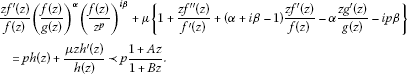(2.5)

Using Lemma 1.4 for $λ= p μ$ and $γ=0$, we have

$h(z)≺q(z)≺ 1 + A z 1 + B z ,$

where $q(z)$ is given in (2.3) and is the best dominant of (2.5). Next, in order to prove $M p (α,β,μ,A,B)⊂ B p (α,β,ρ)$, we show that $inf | z | < 1 {Req(z)}=q(−1)$. Now, we set $a= p μ (B−A)/B$, $b= p μ$ and $c= p μ +1$, then it is clear that $c>b>0$; therefore, for $B≠0$ it follows from (2.2) by using Lemma 1.6 that

$Q ( z ) = ( 1 + B z ) a ∫ 0 1 t b − 1 ( 1 + B t z ) − a d t = Γ ( b ) Γ ( c ) 2 F 1 ( 1 , a , c ; B z B z + 1 ) .$
(2.6)

To prove that $inf | z | < 1 {Req(z)}=q(−1)$, we need to show that

$Re { 1 / Q ( z ) } ≥1/Q(−1).$

Since $A<− μ B p$ with $−1≤B<0$ implies that $c>a>0$, therefore, by using Lemma 1.6, (2.6) yields

$Q(z)= ∫ 0 1 g(z,t)dε(t),$

where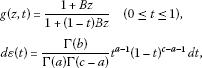which is a positive measure on $[0,1]$. For $−1≤B<0$ it is clear that $Reg(z,t)>0$ and $g(−r,t)$ is real for $0≤|z|≤r<1$ and $t∈[0,1]$. Also,

$Re { 1 g ( z , t ) } =Re { 1 + ( 1 − t ) B z 1 + B z } ≥ 1 − ( 1 − t ) B r 1 − B r = 1 g ( − r , t )$

for $|z|≤r<1$. Therefore, using Lemma 1.5, we have

$Re { 1 / Q ( z ) } ≥1/Q(−r).$

Now, letting $r→ 1 −$, it follows

$Re { 1 / Q ( z ) } ≥1/Q(−1).$

Therefore, $M p (α,β,μ,A,B)⊂ B p (α,β,ρ)$. □

For $β=0$, we have the following result proved in .

Corollary 2.2 If $f∈ M p (α,μ,A,B)$, then

$z f ′ ( z ) p f ( z ) ( f ( z ) g ( z ) ) α ≺{ [ 2 F 1 ( 1 , p μ ( 1 − A B ) ; p μ + 1 ; B z B z + 1 ) ] − 1 , B ≠ 0 , [ 1 F 1 ( 1 , p μ + 1 ; − p μ A z ) ] − 1 , B = 0 ,$

and if $A<− μ B p$, $−1≤B<0$, then $M p (α,μ,A,B)⊂ B p (α,ρ)$, where

$ρ=p { 2 F 1 ( 1 , p μ ( 1 − A B ) ; p μ + 1 ; B B − 1 ) } − 1 .$

This result is best possible.

For $p=1$, we have the class $M 1 (α,β,μ,A,B)=M(α,β,μ,A,B)$. We denote the class of functions $f∈A$, having Taylor series representation of the form

$f(z)=z+ ∑ k = n + 1 ∞ a k z k$

and satisfying the condition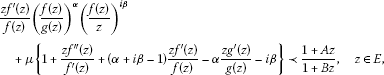(2.7)

by $M ∗ (α,β,μ,A,B)$, where $g(z)=z+ ∑ k = n + 1 ∞ b k z k$ such that $Re z g ′ ( z ) g ( z ) >0$. Now, we derive the following result for the class $M ∗ (α,β,μ,A,B)$.

Theorem 2.3 Let $f∈ M ∗ (α,β,μ,A,B)$. Then

$| a n + 1 |≤ ( A − B ) + α ( n + 1 ) ( 1 + μ n ) | α + i β + n | ( 1 + μ n ) .$
(2.8)

Proof Since $f∈ M ∗ (α,β,μ,A,B)$, therefore,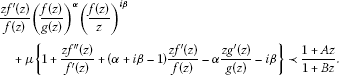Now, using the fact that $f(z)=z+ ∑ k = n + 1 ∞ a k z k$ and $g(z)=z+ ∑ k = n + 1 ∞ b k z k$, we obtain

$1+ { ( α + i β + n ) ( 1 + μ n ) a n + 1 − α ( 1 + μ n ) b n + 1 } z n +⋯≺ 1 + A z 1 + B z .$

By a well-known result due to Janowski and Lemma 1.9, we have

$|(α+iβ+n)(1+μn) a n + 1 −α(1+μn) b n + 1 |≤A−B.$

By the triangle inequality, we obtain

$|(α+iβ+n)(1+μn) a n + 1 |−|α(1+μn) b n + 1 |≤A−B.$

Using the coefficient bound for the class $S ∗$, we have the required result. □

For $β=0$ and $g(z)=z$, we have the following result proved in .

Corollary 2.4 Let $f∈M(α,μ,A,B)$. Then

$| a n + 1 |≤ ( A − B ) ( α + n ) ( 1 + μ n ) .$

For $β=0$, $p=1$, $g(z)=z$, $A=1−2ρ$ and $B=−1$, we have the following result proved in .

Corollary 2.5 Let f satisfy the condition

$Re { z f ′ ( z ) f ( z ) ( f ( z ) z ) α + μ [ 1 + z f ′ ′ ( z ) f ′ ( z ) + ( 1 − α ) ( 1 − z f ′ ( z ) f ( z ) ) ] } >ρ.$

Then

$| a n + 1 |≤ 2 ( 1 − ρ ) ( n + α ) ( 1 + μ n ) .$

Theorem 2.6 For $μ 2 ≥ μ 1 ≥0$ and $−1≤ B 1 ≤ B 2 < A 2 ≤ A 1 ≤1$,

$M p (α,β, μ 2 , A 2 , B 2 )⊂ M p (α,β, μ 1 , A 1 , B 1 ).$

Proof Let $f∈ M p (α,β, μ 2 , A 2 , B 2 )$. Then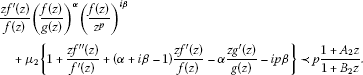Since $−1≤ B 1 ≤ B 2 < A 2 ≤ A 1 ≤1$, therefore by Lemma 1.7, we have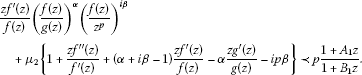Hence, we have $f∈ M p (α,β, μ 2 , A 1 , B 1 )$. For $μ 2 = μ 1 ≥0$, we have the required result. When $μ 2 > μ 1 ≥0$, Theorem 2.1 implies that

$z f ′ ( z ) f ( z ) ( f ( z ) g ( z ) ) α ( f ( z ) z p ) i β ≺p 1 + A 1 z 1 + B 1 z .$

NowUsing Lemma 1.8, we have the required result. □

For $β=0$, we have the following result.

Corollary 2.7 For $μ 2 ≥ μ 1 ≥0$ and $−1≤ B 1 ≤ B 2 < A 2 ≤ A 1 ≤1$,

$M p (α, μ 2 , A 2 , B 2 )⊂ M p (α, μ 1 , A 1 , B 1 ).$

This result is proved in .

For $β=0$, $p=1$, $g(z)=z$, $A=1−2ρ$ and $B=−1$, we have the class $M(α,μ,ρ)$ defined as

$Re [ z f ′ ( z ) f ( z ) ( f ( z ) z ) α + μ { 1 + z f ′ ′ ( z ) f ′ ( z ) − z f ′ ( z ) f ( z ) + α ( z f ′ ( z ) f ( z ) − 1 ) } ] >ρ$
(2.9)

for $z∈E$. Now have the following result for the class $M(α,μ,ρ)$ proved in .

Corollary 2.8 For $α≥0$, $μ 2 ≥ μ 1 ≥0$ and $1> ρ 2 ≥ ρ 1 ≥0$,

$M(α, μ 2 , ρ 2 )⊂M(α, μ 1 , ρ 1 ).$

Theorem 2.9 Let $f∈ A p$ satisfy

$Re { f ( z ) z p } >0and| z f ′ ( z ) f ( z ) ( f ( z ) g ( z ) ) α ( f ( z ) z p ) i β −p|<σp,0<σ≤1,$

for $g∈ S p ∗$. Then f is p-valent convex in $|z|< R α , β , σ$, where

$R α , β , σ = ( ( 2 | 1 − α − i β | + 2 α p + σ ) − ( 2 | 1 − α − i β | + 2 α p + σ ) 2 − 4 p ( 2 α p − p − σ ) ) / ( 2 ( 2 α p − p − σ ) ) .$
(2.10)

Proof Let

$h(z)= z f ′ ( z ) p f ( z ) ( f ( z ) g ( z ) ) α ( f ( z ) z p ) i β −1,$

where $h(z)$ is analytic in E with $h(0)=0$ and $|h(z)|<1$. By using the Schwarz lemma, we get

$h(z)=σzψ(z),$

where $ψ(z)$ is analytic in E with $|ψ(z)|<1$. Differentiating logarithmically, we have

$1+ z f ′ ′ ( z ) f ′ ( z ) =(1−α−iβ) z f ′ ( z ) f ( z ) +α z g ′ ( z ) g ( z ) + σ z ( z ψ ′ ( z ) + ψ ( z ) ) 1 + σ z ψ ( z ) +ipβ.$

Since $Re{ f ( z ) z p }>0$, therefore,

$z f ′ ( z ) f ( z ) =p+ z φ ′ ( z ) φ ( z ) ,Reφ(z)>0.$

This implies that

$Re { 1 + z f ′ ′ ( z ) f ′ ( z ) } ≥(1−α)p+αRe z g ′ ( z ) g ( z ) −|1−α−iβ|| z φ ′ ( z ) φ ( z ) |−σ| z ( z ψ ′ ( z ) + ψ ( z ) ) 1 + σ z ψ ( z ) |.$

Now, using the well-known results for classes $S p ∗$, P and the Schwarz function , we have

$Re { 1 + z f ′ ′ ( z ) f ′ ( z ) } ≥ ( 1 − α ) p + α p 1 − r 1 + r − | 1 − α − i β | 2 r ( 1 − r 2 ) − σ r ( 1 − r ) ( 0 < σ ≤ 1 ) = ( 2 α p − p − σ ) r 2 − ( 2 | 1 − α − i β | + 2 α p + σ ) r + p ( 1 − r 2 ) .$

Let $P(r)=(2αp−p−σ) r 2 −(2|1−α−iβ|+2αp+σ)r+p$. Since $p∈N$ and $0<σ≤1$, therefore, $P(0)=p>0$ and $P(1)=−2(|1−α−iβ|+σ)<0$. It follows that the root lies in $(0,1)$. This implies that $Re{1+ z f ′ ′ ( z ) f ′ ( z ) }>0$ if $r< R α , β , σ$, where $R α , β , σ$ is given by (2.10). □

For $σ=1$ and $β=0$, we have the following result which is proved in .

Corollary 2.10 Let $f∈ A p$ satisfy

$Re { f ( z ) z p } >0and| z f ′ ( z ) f ( z ) ( f ( z ) g ( z ) ) α −p|

$g∈ S p ∗$. Then f is p-valent convex in $|z|< R α$, where

$R α = 3 + 2 α ( p − 1 ) − ( 3 + 2 α ( p − 1 ) ) 2 − 4 p ( 2 α p − p − 1 ) 2 ( 2 α p − p − 1 ) .$

Theorem 2.11 Let $f∈ A p$ satisfy

$| z f ′ ( z ) f ( z ) ( f ( z ) g ( z ) ) α −p|<σp,$

for $g∈ S p ∗$. Then, for $α>0$, f is p-valent $1 α$-convex in $|z|< R α , σ$, where

$R α , σ = 2 α p + σ − ( 2 α p + σ ) 2 − 4 α p ( α p − σ ) 2 ( α p − σ ) .$
(2.11)

Proof Let

$h(z)= z f ′ ( z ) p f ( z ) ( f ( z ) g ( z ) ) α −1,$

where $h(z)$ is analytic in E with $h(0)=0$ and $|h(z)|<1$. By using the Schwarz lemma, we get

$h(z)=σzψ(z),$

where $ψ(z)$ is analytic in E with $|ψ(z)|<1$. Differentiating logarithmically, we have

$1 α ( 1 + z f ′ ′ ( z ) f ′ ( z ) ) + ( 1 − 1 α ) z f ′ ( z ) f ( z ) = z g ′ ( z ) g ( z ) + σ α z ( z ψ ′ ( z ) + ψ ( z ) ) 1 + σ z ψ ( z ) .$

This implies that

$Re { 1 α ( 1 + z f ′ ′ ( z ) f ′ ( z ) ) + ( 1 − 1 α ) z f ′ ( z ) f ( z ) } ≥Re z g ′ ( z ) g ( z ) − σ α | ( z ψ ′ ( z ) + ψ ( z ) ) 1 + σ z ψ ( z ) |.$

Now, using the well-known results for classes $S p ∗$, and the Schwarz function, we have

$Re 1 p { 1 α ( 1 + z f ′ ′ ( z ) f ′ ( z ) ) + ( 1 − 1 α ) z f ′ ( z ) f ( z ) } ≥ 1 − r 1 + r − σ r α p ( 1 − r ) ( 0 < σ ≤ 1 ) = ( α p − σ ) r 2 − ( 2 α p + σ ) r + α p α ( 1 − r 2 ) .$

Let $Q(r)=(αp−σ) r 2 −(2αp+σ)r+αp$. Then for $p∈N$, $α>0$ and $0<σ≤1$, $Q(0)=αp>0$ and $Q(1)=−2σ<0$. It shows that the root lies in $(0,1)$. This implies that $Re{ 1 α (1+ z f ′ ′ ( z ) f ′ ( z ) )+(1− 1 α ) z f ′ ( z ) f ( z ) }>0$ if $r< R α , σ$, where $R α , σ$ is given by (2.11). □

Theorem 2.12 Let $f∈M(α,β,μ,A,B)$. Then E is mapped by f on a domain that contains the disc $|w|< R α , β , μ ∈(0,1)$, where

$R α , β , μ = | α + i β + 1 | ( 1 + μ ) 2 | α + i β + 1 | ( 1 + μ ) + ( A − B ) + 2 α ( 1 + μ ) .$
(2.12)

Proof Let $w 0$ be any complex number such that $f(z)≠ w 0$. Then

$w 0 f ( z ) w 0 − f ( z ) =z+ ( a 2 + 1 w 0 ) z 2 +⋯,$

is univalent in E, so that

$| a 2 + 1 w 0 |≤2.$

Therefore,

$| 1 w 0 |−| a 2 |≤2.$

Hence,

$w 0 ≥ | α + i β + 1 | ( 1 + μ ) 2 | α + i β + 1 | ( 1 + μ ) + ( A − B ) + 2 α ( 1 + μ ) = R α , β , μ .$

□

For $β=0$, $g(z)=z$, $A=1−2 ρ 1$, $B=−1$, we have the following result proved in .

Corollary 2.13 Let $f∈M(α,μ,ρ)$. Then E is mapped by f on a domain that contains the disc $|w|< R α , μ$, where

$R α , β , μ = ( 1 + α ) ( 1 + μ ) 2 ( 1 + α ) ( 1 + μ ) + 2 ( 1 − ρ 1 ) .$

Theorem 2.14 Let $α>0$, $c≥−α 1 − A 1 − B$ and let $f∈A$. If

$|arg z f ′ ( z ) f ( z ) ( f ( z ) g ( z ) ) α ( f ( z ) z ) i β −ρ|< π 2 υ(0<υ≤1,0≤ρ<1),$
(2.13)

for some $g∈ S ∗$, then

$|arg z F ′ ( z ) F ( z ) ( F ( z ) G ( z ) ) α ( F ( z ) z ) i β −ρ|< π 2 η(0<η≤1),$
(2.14)

where

$F(z)= [ c + α + i β z c ∫ 0 z t c − 1 f α + i β ( t ) d t ] 1 α + i β ,$
(2.15)

with

$υ={ η + 2 π tan − 1 ( ( 1 + B ) η sin ( π ( 1 − t ( α , β , c , A , B ) ) / 2 ) | c + i β | ( 1 + B ) + α ( 1 + A ) + η ( 1 + B ) cos ( π ( 1 − t ( α , β , c , A , B ) ) / 2 ) ) , B ≠ − 1 , η , B = − 1 ,$
(2.16)

and

$t(α,β,c,A,B)= 2 π sin − 1 α ( A − B ) + β ( 1 − B 2 ) | c + i β | ( 1 − B 2 ) + α ( 1 − A B ) .$
(2.17)

Proof Since

$F α + i β (z)= c + α + i β z c ∫ 0 z t c − 1 f α + i β (t)dt,$

therefore,

$z 1 − i β F α + i β − 1 (z) F ′ (z)= 1 α + i β { ( c + α + i β ) z − i β f α + i β ( z ) − c z − i β F α + i β ( z ) } .$

Now using (1.5), we have

$z F ′ ( z ) F ( z ) ( F ( z ) G ( z ) ) α ( F ( z ) z ) i β = 1 α + i β { ( c + α + i β ) z c f α + i β ( z ) − c z c F α + i β ( z ) } ( c + α + i β ) ∫ 0 z t c + i β − 1 g α ( t ) d t .$

Let

$h(z)= 1 1 − ρ ( z F ′ ( z ) F ( z ) ( F ( z ) G ( z ) ) α ( F ( z ) z ) i β − ρ ) = N ( z ) D ( z ) ,$

where $h(z)$ is analytic with $h(0)=1$. Now

$N ′ ( z ) D ′ ( z ) = 1 1 − ρ ( z f ′ ( z ) f ( z ) ( f ( z ) g ( z ) ) α ( f ( z ) z ) i β − ρ ) = h ( z ) { 1 + D ( z ) z D ′ ( z ) ⋅ z h ′ ( z ) h ( z ) } ,$

where

$N ( z ) = 1 1 − ρ ( 1 α + i β { ( c + α + i β ) z c f α + i β ( z ) − c z c F α + i β ( z ) } − ρ z c + i β − 1 G α ( z ) ) , D ( z ) = ( c + α + i β ) ∫ 0 z t c + i β − 1 g α ( t ) d t .$

Since $G∈ S ∗ [A,B]$, therefore, we can write

$z D ′ ( z ) D ( z ) =c+iβ+α z G ′ ( z ) G ( z ) = r 1 e i π 2 θ ,$

where

${ | c + i β | + α 1 − A 1 − B < r 1 < | c + i β | + α 1 + A 1 + B , B ≠ − 1 , − t ( α , β , c , A , B ) < θ < t ( α , β , c , A , B ) , B ≠ − 1 ,$
(2.18)

similarly

${ | c + i β | + α 1 − A 2 < r 1 < ∞ , B = − 1 , − 1 < θ < 1 , B = − 1 .$
(2.19)

Suppose that $h(z)≠0$ in E, there exists a point $z 0 ∈E$ such that $|argh(z)|< π 2 η$ ($|z|<| z 0 |$) and $|argh( z 0 )|= π 2 η$. Now, using Lemma 1.10, we have $z h ′ ( z 0 ) h ( z 0 ) =ikη$. At first suppose that $h( z 0 )= ( i x ) η$ ($x>0$) for the case $B≠−1$, we obtain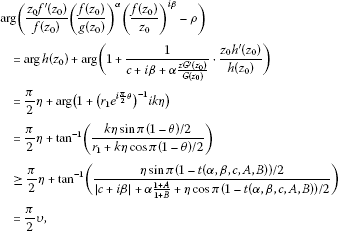where υ and $t(α,β,c,A,B)$ are given by (2.16) and (2.17) respectively. For $B=−1$, we have

$arg ( z 0 f ′ ( z 0 ) f ( z 0 ) ( f ( z 0 ) g ( z 0 ) ) α ( f ( z 0 ) z 0 ) i β − ρ ) ≥ π 2 η,$

which is a contradiction to the assumption of our theorem. Now we suppose that $h( z 0 )= ( − i x ) η$. For the case $B≠−1$ using a similar method, we obtain

$arg ( z 0 f ′ ( z 0 ) f ( z 0 ) ( f ( z 0 ) g ( z 0 ) ) α ( f ( z 0 ) z 0 ) i β − ρ ) ≤− π 2 υ,$

and for $B=−1$, we have

$arg ( z 0 f ′ ( z 0 ) f ( z 0 ) ( f ( z 0 ) g ( z 0 ) ) α ( f ( z 0 ) z 0 ) i β − ρ ) ≤− π 2 η,$

which is a contradiction to the assumption of our theorem. Hence, we have the proof. □

This kind of problem is also considered in . For $β=0$, we have the following result proved in .

Corollary 2.15 Let $α>0$, $c≥−α 1 − A 1 − B$ and let $f∈A$. If

$|arg ( z f ′ ( z ) f ( z ) ( f ( z ) g ( z ) ) α − ρ ) |< π 2 υ(0<υ≤1),$

then

$|arg ( z F ′ ( z ) F ( z ) ( F ( z ) G ( z ) ) α − ρ ) |< π 2 η(0<η≤1),$

where

$F(z)= [ c + α z c ∫ 0 z t c − 1 f α ( t ) d t ] 1 α ,$

with

$υ={ η + 2 π tan − 1 ( ( 1 + B ) η sin ( π ( 1 − t ( α , β , c , A , B ) ) / 2 ) c ( 1 + B ) + α ( 1 + A ) + η ( 1 + B ) cos ( π ( 1 − t ( α , β , c , A , B ) ) / 2 ) ) , B ≠ − 1 , η , B = − 1 ,$

and

$t(α,β,c,A,B)= 2 π sin − 1 α ( A − B ) c ( 1 − B 2 ) + α ( 1 − A B ) .$

Remark 2.16 By using the suitable choices of parameters c, α, A and B, we can find many results proved in the literature.

## References

1. 1.

Çag̃lar M, Polatog̃lu Y, Şen A, Yavuz E, Owa S: On Janowski starlike functions. J. Inequal. Appl. 2007. doi:10.1155/2007/14630

2. 2.

Sokół J: On a class of analytic multivalent functions. Appl. Math. Comput. 2008, 203: 210–216. 10.1016/j.amc.2008.04.027

3. 3.

Sokół J: Classes of multivalent functions associated with a convolution operator. Comput. Math. Appl. 2010, 60: 1343–1350. 10.1016/j.camwa.2010.06.015

4. 4.

Arif M, Noor KI, Raza M: Hankel determinant problem of a subclass of analytic functions. J. Inequal. Appl. 2012. doi:10.1186/1029–242X-2012–22

5. 5.

Arif M, Noor KI, Raza M: On a class of analytic functions related with generalized Bazilevic type functions. Comput. Math. Appl. 2011, 61: 2456–2462. 10.1016/j.camwa.2011.02.026

6. 6.

Arif M, Raza M, Noor KI, Malik SN: On strongly Bazilevic functions associated with generalized Robertson functions. Math. Comput. Model. 2011, 54: 1608–1612. 10.1016/j.mcm.2011.04.033

7. 7.

Bazilevic IE: On a class of integrability in quadratures of the Loewner-Kufarev equation. Mat. Sb. N.S. 1955, 37: 471–476.

8. 8.

El-Ashwah RM, Aouf MK: Some properties of certain classes of meromorphically p -valent functions involving extended multiplier transformations. Comput. Math. Appl. 2010, 59: 2111–2120. 10.1016/j.camwa.2009.12.016

9. 9.

Noor KI: On Bazilevic functions of complex order. Nihonkai Math. J. 1992, 3: 115–124.

10. 10.

Noor KI: Bazilevic functions of type β . Int. J. Math. Math. Sci. 1982, 5(2):411–415. 10.1155/S0161171282000416

11. 11.

Raza M, Noor KI: A class of Bazilevic type functions defined by convolution operator. J. Math. Inequal. 2011, 5(2):253–261.

12. 12.

Patel J: On certain subclass of p -valently Bazilevic functions. J. Inequal. Pure Appl. Math. 2005., 6(1): Article ID 16

13. 13.

Wang Z-G, Jiang Y-P: Notes on certain subclass of p -valently Bazilevic functions. J. Inequal. Pure Appl. Math. 2008., 9(3): Article ID 70

14. 14.

Miller SS, Mocanu PT: Univalent solutions of Briot-Bouquet differential subordination. J. Differ. Equ. 1985, 56: 297–309. 10.1016/0022-0396(85)90082-8

15. 15.

Wilken DR, Feng J: A remark on convex and starlike functions. J. Lond. Math. Soc. 1980, 21: 287–290. 10.1112/jlms/s2-21.2.287

16. 16.

Whittaker ET, Watson GN: A Course of Modern Analysis. 4th edition. Cambridge University Press, Cambridge; 1958.

17. 17.

Liu M-S: On a subclass of p -valent close-to-convex functions of order β and type α . J. Math. Study 1997, 30: 102–104. in Chinese

18. 18.

Liu M-S: On certain subclass of analytic functions. J. South China Norm. Univ. 2002, 4: 15–20. in Chinese

19. 19.

Rogosinski W: On coefficient of subordinate functions. Proc. Lond. Math. Soc. 1943, 48: 48–82.

20. 20.

Nunokawa M: On the order of strongly starlikeness of strongly convex functions. Proc. Jpn. Acad., Ser. A, Math. Sci. 1993, 68: 234–237.

21. 21.

Guo D, Liu M-S: On certain subclass of Bazilevic functions. J. Inequal. Pure Appl. Math. 2007., 8(1): Article ID 12

22. 22.

Causey WM, Merkes EP: Radii of starlikeness for certain classes of analytic functions. J. Math. Anal. Appl. 1970, 31: 579–586. 10.1016/0022-247X(70)90010-7

23. 23.

El-Ashwah RM: Results regarding the argument of certain p -valent analytic functions defined by a generalized integral operator. J. Inequal. Appl. 2012., 2012: Article ID 35. doi:10.1186/1029–242X-2012–35

24. 24.

Cho NE, Kim IH, Kim JA: Angular estimation of certain integral operators. Int. J. Math. Math. Sci. 1998, 21(2):369–374. 10.1155/S0161171298000507

## Acknowledgements

The third author would like to express gratitude to Dr. S.M. Junaid Zaidi, Rector CIIT for his support and for providing excellent research facilities and to the Higher Education Commission of Pakistan for financial assistance.

## Author information

Authors

### Corresponding author

Correspondence to Mohsan Raza.

### Competing interests

The authors declare that they have no competing interests.

### Authors’ contributions

MR, SNM and KIN jointly discussed and presented the ideas of this article. MR made the text file and all the communications regarding the manuscript. All authors read and approved the final manuscript.

## Rights and permissions

Reprints and Permissions

Raza, M., Malik, S.N. & Noor, K.I. On some inequalities of a certain class of analytic functions. J Inequal Appl 2012, 250 (2012). https://doi.org/10.1186/1029-242X-2012-250